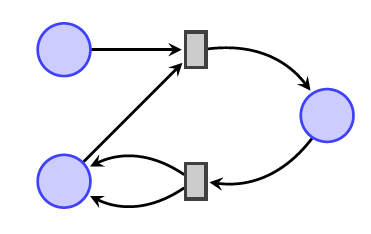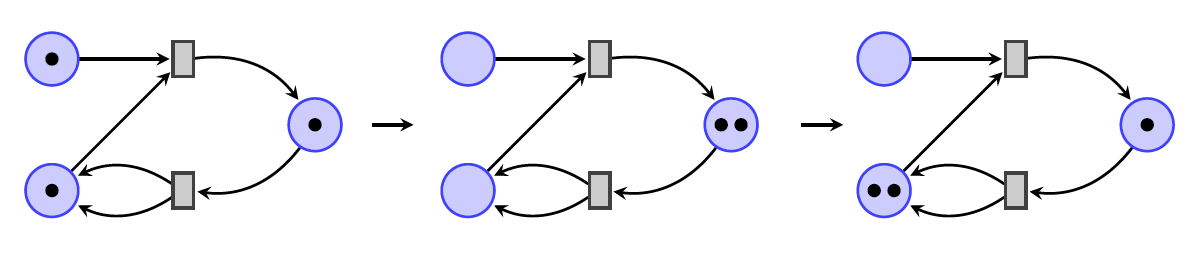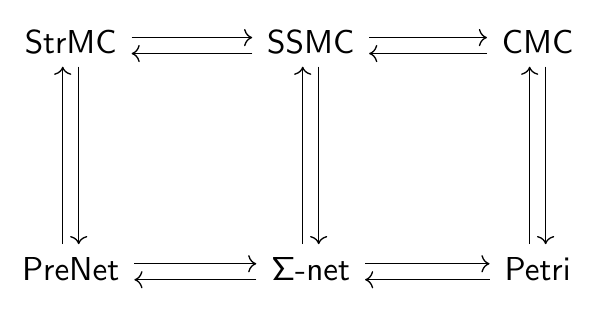## January 17, 2021

### Categories of Nets (Part 1)

#### Posted by John BaezI’ve been thinking about Petri nets a lot. Around 2010, I got excited about using them to describe chemical reactions, population dynamics and more, using ideas taken from quantum physics. Then I started working with my student Blake Pollard on ‘open’ Petri nets, which you can glue together to form larger Petri nets. Blake and I focused on their applications to chemistry, but later my student Jade Master and I applied them to computer science and brought in some new math. I was delighted when Evan Patterson and Micah Halter used all this math, along with ideas of Joachim Kock, to develop software for rapidly assembling models of COVID-19.

Now I’m happy to announce that Jade and I have teamed up with Fabrizio Genovese and Mike Shulman to straighten out a lot of mysteries concerning Petri nets and their variants:

This paper is full of interesting ideas, but I’ll just tell you the basic framework.

A Petri net is a seemingly simple thing:It consists of places (drawn as circles) and transitions (drawn as boxes), with directed edges called arcs from places to transitions and from transitions to places.

The idea is that when you use a Petri net, you put dots called tokens in the places, and then move them around using the transitions:A Petri net is actually a way of describing a monoidal category. A way of putting a bunch of tokens in the places gives an object of this category, and a way of moving them around repeatedly (as above) gives a morphism.

The idea sounds straightforward enough. But it conceals some subtleties, which researchers have been struggling with for at least 30 years.

There are various ways to make the definition of Petri net precise. For example: is there a finite set of arcs from a given place to a given transition (and the other way around), or merely a natural number of arcs? If there is a finite set, is this set equipped with an ordering or not? Furthermore, what is a morphism between Petri nets?

Different answers are good for different purposes. In the so-called ‘individual token philosophy’, we allow a finite set of tokens in each place. In the ‘collective token philosophy’, we merely allow a natural number of tokens in each place. It’s like the difference between having 4 individual workers named John, Fabrizio, Jade and Mike where you can tell who did what, and having 4 anonymous workers: nameless drones.

Our goal was to sort this out all and make it crystal clear. We focus on 3 kinds of net, each of which naturally generates its own kind of monoidal category:

• pre-nets, which generate free strict monoidal categories.
• Σ-nets, which generate free symmetric strict monoidal categories.
• Petri nets, which generate free commutative monoidal categories.

These three kinds of monoidal category differ in how ‘commutative’ they are:

• In a strict monoidal category we typically have $x \otimes y \ne y \otimes x$.

• In a strict symmetric monoidal category we have for each pair of objects a chosen isomorphism $x \otimes y \cong y \otimes x$.

• A commutative monoidal category is a symmetric strict monoidal category where the symmetry isomorphisms are all identities, so $x \otimes y = y \otimes x$.

So, we have a spectrum running from hardcore individualism, where two different things of the same type are never interchangeable… to hardcore collectivism, where two different things of the same type are so interchangeable that switching them counts as doing nothing at all! In the theory of Petri nets and their variants, the two extremes have been studied better than the middle.

You can summarize the story with this diagram:There are three different categories of nets at bottom, and three diffferent categories of monoidal categories on top — all related by adjoint functors! Here the left adjoints point up the page — since different kinds of nets freely generate different kinds of monoidal categories — and also to the right, in the direction of increasing ‘commutativity’.

If you’re a category theorist you’ll recognize at least two of the three categories on top:

• $\mathsf{StrMC}$, with strict monoidal categories as objects and strict monoidal functors as morphisms.

• $\mathsf{SSMC}$, with symmetric strict monoidal categories as objects and strict symmetric monoidal functors as their morphisms.

• $\mathsf{CMC}$, with commutative monoidal categories as objects and strict symmetric monoidal functors as morphisms. A commutative monoidal category is a symmetric strict monoidal category where the symmetry is the identity.

The categories of nets are probably less familiar. But they are simple enough. Here I’ll just describe their objects. The morphisms are fairly obvious, but read our paper for details.

• $\mathsf{PreNet}$, with pre-nets as objects. A pre-net consists of a set $S$ of places, a set $T$ of transitions, and a function $T \to S^\ast\times S^\ast$, where $S^\ast$ is the underlying set of the free monoid on $S$.

• Σ$\mathsf{-net}$, with Σ-nets as objects. A Σ-net consists of a set $S$, a groupoid $T$, and a discrete opfibration $T \to P S \times P S^{\mathrm{op}}$, where $P S$ is the free symmetric strict monoidal category generated by a set of objects $S$ and no generating morphisms.

• $\mathsf{Petri}$, with Petri nets as objects. A Petri net, as we use the term, consists of a set $S$, a set $T$, and a function $T \to \mathbb{N}[S] \times \mathbb{N}[S]$, where $\mathbb{N}[S]$ is the underlying set of the free commutative monoid on $S$.

What does this mean in practice?

• In a pre-net, each transition has an ordered list of places as ‘inputs’ and an ordered list of places as ‘outputs’. We cannot permute the inputs or outputs of a transition.

• In a Σ-net, each transition has an ordered list of places as inputs and an ordered list of places as outputs. However, permuting the entries of these lists gives a new transition with a new list of inputs and a new list of outputs!

• In a Petri net, each transition has a multiset of places as inputs and a multiset of places as outputs. A multiset is like an ‘unordered list’: entries can appear repeatedly, but the order makes no difference at all.

So, pre-nets are rigidly individualist. Petri nets are rigidly collectivist. And Σ-nets are flexible, including both extremes as special cases!

On the one hand, we can use the left adjoint functor

$\mathsf{PreNet} \to \Sigma\mathsf{-net}$

to freely generate Σ-nets from pre-nets. If we do this, we get Σ-nets such that permutations of inputs and outputs act freely on transitions. Joachim Kock has recently studied Σ-nets of this sort. He calls them whole-grain Petri nets, and he treats them as forming a category in their own right, but it’s also the full image of the above functor.

On the other hand, we can use the right adjoint functor

$\mathsf{Petri} \to Σ\mathsf{-net}$

to turn Petri nets into Σ-nets. If we do this, we get Σ-nets such that permutations of inputs and outputs act trivially on transitions: the permutations have no effect at all.

I’m not going to explain how we got any of the adjunctions in this diagram:That’s where the interesting category theory comes in. Nor will I tell you about the various alternative mathematical viewpoints on Σ-nets… nor how we draw them. I also won’t explain our work on open nets and open categories of all the various kinds. I’m hoping Mike Shulman will say some more about what we’ve done. That’s why this blog article is optimistically titled “Part 1”.

But I hope you see the main point. There are three different kinds of things like Petri nets, each of which serves to freely generate a different kind of monoidal category. They’re all interesting, and a lot of confusion can be avoided if we don’t mix them up!

Posted at January 17, 2021 8:49 PM UTC

TrackBack URL for this Entry:   https://golem.ph.utexas.edu/cgi-bin/MT-3.0/dxy-tb.fcgi/3287

### Re: Categories of Nets (Part 1)

Interesting!

Your final paragraph on group actions, moduli spaces and orbifolds put me in mind of Urs’s treatment of singularities in his paper with Hisham Sati, the ‘orbi-singularity’ mediating between the singular quotient and the smooth homotopy quotient.

Posted by: David Corfield on January 18, 2021 8:07 AM | Permalink | Reply to this

### Re: Categories of Nets (Part 1)

Which might make one think, with all those adjunctions about, that some modal version of Mike’s practical type theory for symmetric monoidal categories could be of use.

Posted by: David Corfield on January 18, 2021 8:54 AM | Permalink | Reply to this

### Re: Categories of Nets (Part 1)

The “practical type theory” paper is essentially a syntactic and “minimal quotienting” way of presenting the free SSMC generated by a pre-net. I assume that it could be adapted to generate an SSMC from a $\Sigma$-net too, although there would be more quotienting.

Posted by: Mike Shulman on January 19, 2021 5:37 PM | Permalink | Reply to this

### Re: Categories of Nets (Part 1)

The firing rule wasn’t made quite explicit in the first example you gave (which is also the one in your Arxiv preprint). I believe it is: a transition gate may fire when there is at least one token available for each of its input arrows; when it fires it consumes one token from each input arrow, and sends one token along each of its output arrows and these two number of tokens do not have to be the same. Is that correct?

Posted by: Richard Pinch on January 18, 2021 10:57 AM | Permalink | Reply to this

### Re: Categories of Nets (Part 1)

Yes, that’s exactly correct!

This “firing rule” is standard in Petri net theory. In our paper, it’s deeply buried in our recipes for getting categories from Petri nets: it’s there implicitly, but it would take quite a bit of thought to ferret it out. That’s a bit unfortunate, but I guess we’re so used to it that we didn’t think of explaining it.

In my earlier paper with Jade:

• John Baez and Jade Master, Open Petri nets, Mathematical Structures in Computer Science 30 (2020), 314–341.

it’s spelled out explicitly in Definition 20. A marking of a Petri net is a way of labeling each place with a natural number: think of it as the number of tokens in that place. We then say when it’s possible for a transition to fire, and how the marking changes when this happens.

It’s just what you said.

Posted by: John Baez on January 19, 2021 6:40 AM | Permalink | Reply to this

### Re: Categories of Nets (Part 1)

Posted by: Richard Pinch on January 19, 2021 4:47 PM | Permalink | Reply to this

### Re: Categories of Nets (Part 1)

Of course, for a pre-net or a $\Sigma$-net, a marking should instead label each place by a finite set of tokens, possibly ordered, rather than just a natural number of them, and a firing should specify which tokens are input and output.

Posted by: Mike Shulman on January 19, 2021 5:36 PM | Permalink | Reply to this

### Re: Categories of Nets (Part 1)

I was struck by the analogy with the theory of chip-firing on (undirected) graphs. Obviously any graph chip-firing model can be turned into a particular type of Petri net (the big restriction being “conservation of tokens”). However, one not-at-all obvious thing about the graph model that that there is an abelian group structure defined combinatorially on certain assignments, and the order of this group is equal the number of spanning trees. Is there a similar algebraic structure on the assignments of token numbers to the places of a Petri net?

Posted by: Richard Pinch on January 22, 2021 10:58 AM | Permalink | Reply to this

### Re: Categories of Nets (Part 1)

Richard wrote:

However, one not-at-all obvious thing about the graph model that that there is an abelian group structure defined combinatorially on certain assignments, and the order of this group is equal the number of spanning trees.

Which are “certain” assignments?

I should probably know the answer… it could be lurking in this paper of mine, which I need to read again.

Hmm, if $X$ is a finite graph without bridges, the space $\mathrm{C}_1(X,\mathbb{R})$ of real linear combinations of edges of $X$ gets an inner product where edges form an orthonormal basis, and then the subspace $\mathbb{Z}_1(X,\mathbb{R})$ of 1-cycles inherits an inner product from that, and then the lattice $\mathbb{Z}_1(X,\mathbb{Z})$ of integral cycles is an integral lattice, meaning that the inner product of any two elements is an integer. Thus, we get

$\mathbb{Z}_1(X,\mathbb{Z}) \subseteq \mathbb{Z}_1(X,\mathbb{Z})^\ast$

where the dual lattice $\mathbb{Z}_1(X,\mathbb{Z})^\ast$ consists of all vectors in $\mathbb{Z}_1(X,\mathbb{R})$ whose inner product with everyone in $\mathbb{Z}_1(X,\mathbb{Z})$ is an integer. And then comes the cool part, which goes back at least to the work of Bacher, de la Harpe and Nagnibeda: the order of the quotient group

$\mathbb{Z}_1(X,\mathbb{Z})^\ast/\mathbb{Z}_1(X,\mathbb{Z})$

is the number of spanning trees in $X$.

Posted by: John Baez on January 22, 2021 8:27 PM | Permalink | Reply to this

### Re: Categories of Nets (Part 1)

That does indeed give a natural additive group structure and the Matrix-Tree theorem says that the order of the quotient, which is the order of a codimension-1 minor, is the number of spanning trees.

But the combinatorial construction is intriguingly different. The elements of the group are indeed the configurations of assignments of tokens to vertices, but only those with non-negative values and satisfying a “stability” condition; the group law is addition followed by “stabilisation”; and the group identity is not the all-zero configuration (which indeed is not usually a group element). Stable and stabilisation are concepts coming in various versions involving firing rules which I won’t attempt to get right here: see the paper of Norman Biggs in Journal of Algebraic Combinatorics 9 (1999), 25–45 for one such version.

My point though is that this version of the group is obtained entirely using natural numbers of tokens, and hence has a combinatorial, possibly even a categorical, flavour that might carry across to the more general setting of Petri nets.

Posted by: Richard Pinch on January 22, 2021 10:05 PM | Permalink | Reply to this

### Re: Categories of Nets (Part 1)

Richard wrote:

Is there a similar algebraic structure on the assignments of token numbers to the places of a Petri net?

The assignments of token numbers to the places of a Petri net—called markings for short—form a commutative monoid, meaning you can add them. But the interesting part is that the markings are the objects of a commutative monoidal category!

In other words, not only is there a commutative monoid of markings, but also a commutative monoid of ‘morphisms between markings’, which are roughly ways to go between marking by repeatedly firing transitions. This is the content of Section 2 here:

• John Baez and Jade Master, Open Petri nets, Mathematical Structures in Computer Science 30 (2020), 314–341.

Even better, the resulting functor from the category of Petri nets to the category of commutative monoidal categories is a left adjoint.

(I know I mentioned this paper before, but one can never advertise the work of one’s grad students often enough—or, come to think of it, one’s own.)

Posted by: John Baez on January 22, 2021 8:36 PM | Permalink | Reply to this

Post a New Comment